# Chapter Notes: Quadratic Equations - Notes | Study Mathematics (Maths) Class 10 - Class 10

 Table of contentsQuadratic Equations1 Crore+ students have signed up on EduRev. Have you?

A quadratic equation in the variable x is an equation of the form ax2 + bx + c = 0, where a, b, c are real numbers and a ≠ 0 is called the standard form of a quadratic equation.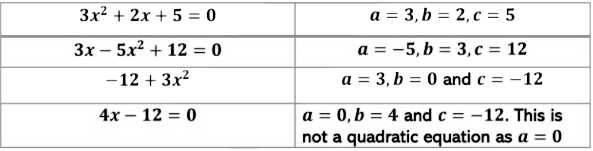Example: Check whether the following are quadratic equations

i) (x + 1)2 = 2(x − 3)
(x + 1)2 = x2 + 2x + 1 ∵ (a + b)2 = a2 + 2ab + b2

x2 + 2x + 1 = 2(x − 3) ⇒ x2 + 2x + 1 = 2x − 6

x2 + 2x + 1 − 2x + 6 = 0 ⇒ x2 + 2x − 2x + 6 + 1 = 0
x2 + 7 = 0
The above equation is a quadratic equation, where the coefficient of x is zero, i.e. b = 0

ii) x(x + 1)(x + 8) = (x + 2)(x − 2)
LHS
x(x + 1)(x + 8) = x(x2 + 8x + x + 8)

= x(x2 + 9x + 8) = x3 + 9x2 + 8x

RHS
(x + 2)(x − 2) = x2 − 4 ∵ (a + b)(a − b) = a2 − b2
Now, x3 + 9x2 + 8x = x2 − 4
x3 + 9x2 − x2 + 8x + 4 = 0
x3 + 8x2 + 8x + 4 = 0
It is not a quadratic equation as it is an equation of degree 3.

iii) (x − 2)+ 1 = 2x − 3
LHS
(x − 2)2 + 1 = x2 − 2x + 4 + 1
∵ (a − b)2 = a2 − 2ab + b2
= x2 − 2x + 5
RHS
2x − 3
x2 − 2x + 5 = 2x − 3
x2 − 2x − 2x + 5 + 3 = 0
x2 − 4x + 8 = 0
The above equation is quadratic as it is of the form,
ax2 + bx + c = 0

Example: The product of two consecutive positive integers is 420. Form the equation satisfying this scenario.

Let the two consecutive positive integers be x and x + 1 Product of the two consecutive integers= x(x + 1) = 420

⇒ x2 + x = 420

x+ x − 420 = 0

x2 + x − 420 = 0, is the required quadratic equation and the two integers satisfy this quadratic equation. Example: A train travels a distance of 480 km at a uniform speed. If the speed had been 8 km/hr less, then it would have taken 4 hr more to cover the distance. We need to find the speed of the train. Form the equation
satisfying this scenario
Let the speed of the train be x km/hr
Distance travelled by train = 480 km = 480/x hr.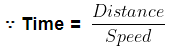If the speed was 8 km/hr less, i.e. (x − 8)km/hr, then the time taken for travelling 480 km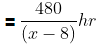According to the question,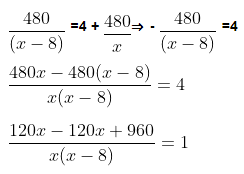960 = x(x-8) ⇒ x2 - 8x - 960 = 0
x2 − 8x − 960 = 0
x2 − 8x − 960 = 0, is the required quadratic equation and the speed of the train satisfies the equation

Solution of Quadratic Equation by Factorisation
A real number α is called a root of the quadratic equation x+bx + c = 0 , a ≠ 0 if aα2 + bα + c = 0
We say that x = α is a solution of the quadratic equation.
Example: x2 − 2x − 3 = 0
If we put x = −1 in the LHS of the above equation we get,
(−1)2 − 2(−1) − 3
1 + 2 − 3 = 0
Thus x = −1 is a solution of the equation x2 − 2x − 3 = 0.
To find the roots of the quadratic equations we follow these steps.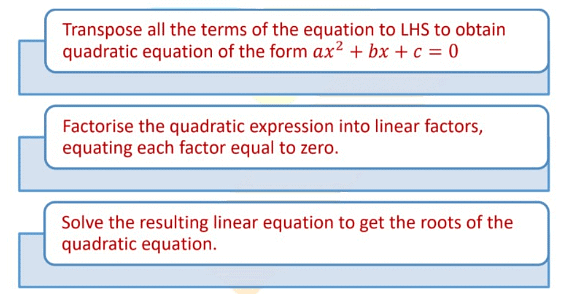Example: Find the roots of the equation x2 − 3x − 10 = 0

The given equation is x2 − 3x − 10 = 0.
Here a = 1, b = −3 and c = −10
1) Find the product of a and c .
Here, the product of a and c= -10 → (ac) is negative

2) Write the factors of this product (ac) such that the sum of the two factors is equal to b.
∴ ac = m × n and m + n = b
Factors of 10 = 2×5
Let m = −5 and n = 2 → (ac=-10)
We write the given equation as,

x2 − 5x + 2x − 10 = 0
x(x − 5) + 2(x − 5) = 0
x − 5 x + 2 = 0
Equate each factor to zero to get the roots of the equation.
x − 5 = 0 and x + 2 = 0
x = 5, −2
Therefore, 5 and -2 are the roots of the equation x
2 − 3x − 10 = 0

Example: Solve the following quadratic equation by factorisation method.

i) 4√3x2  + 5x − 2√3  = 0
The given equation is 4√3x2  + 5x − 2√3  = 0

Here, a = 4√3 , b = 5andc = −2√3

The product of a and c = 4√3  × (−2√3 ) = −8 × 3 = −24
Factors of 24 = 3×8 and 8 + (−3) = 5
The factors of the equation are 8, − 3
So, the given equation can be written as,

4√3x2  + (8 − 3)x − 2√3  = 0 ⇒ 4 √3x2 + 8x − 3x − 2√3  = 0

4x( √3x + 2) −√3 (√3 x + 2) = 0 ⇒ (4x − √3 )(√3x + 2) = 0
Equating each factor to zero we get,

(4x −√3 )=0 and (√3x + 2) = 0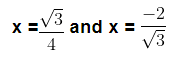The roots of the equation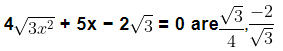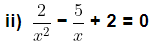The given equation is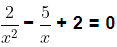Multiplying the above equation by xwe get,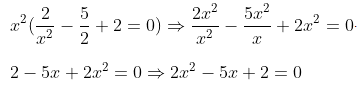Here, a = 2, b = −5 and c = 2
The product of a and c = 2 × 2 = 4
The factors of 4 = 4 × 1 and 4 + 1 = 5
2x2 − (4 + 1)x + 2 = 0 ⇒ 2x2 − 4x − 1x + 2 = 0
2x(x − 2) − (x − 2) = 0
(2x − 1)(x − 2) = 0
Equating each factor to zero we get,
(2x − 1) = 0 and (x − 2) = 0
x =  1/2 and x = 2

The roots of equation 2x− 5x + 2 = 0 are  1/2  and 2

Example: The altitude of a right-angled triangle is 7 cm less than its base. If the hypotenuse is 13 cm long, then find the other two sides.

Let the length of the base be x cm, then altitude = x − 7 cm

Hypotenuse = 13 cm

We know, H2 = P2 + B2
132 = (x − 7)2 + x2 ⇒ 169 = x2 − 14x + 49 + x2
x2 − 14x + 49 + x2 = 169 ⇒ 2x2 − 14x + 49 − 169 = 0
2x2 − 14x − 120 = 0
Dividing the above equation by 2 we get,

x2 − 7x − 60 = 0
Here, a = 1, b = −7 and c = −60
The product of a and c = 1 × (−60) = −60
The factors of 60 = 5 × 12 and −12 + 5 = 7
The given equation can be written as,

x2 − 12x + 5x − 60 = 0
x(x − 12) + 5(x − 12) = 0 ⇒ (x + 5)(x − 12) = 0
Equating each factor to zero we get,
(x + 5) = 0 and (x − 12) = 0 ⇒ x = −5 and x = 12
The length of the base cannot be negative.
Therefore, Base = 12 cm
Altitude = x − 7 cm = 12 − 7 = 5 cm, Hypotenuse = 13 cm

Solution of the Quadratic Equations by Completing the  Square

If we have to find the solution of a quadratic equation by completing the square, we follow the steps given below.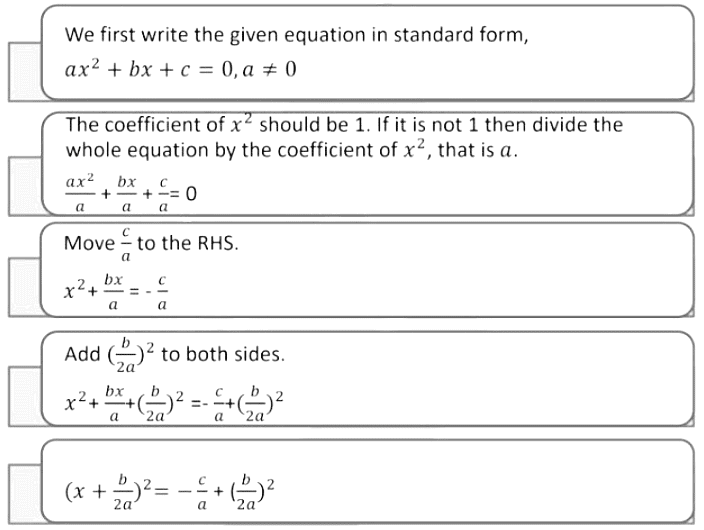The complete square is,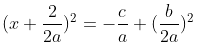Let’s learn to complete the square with the help of a diagram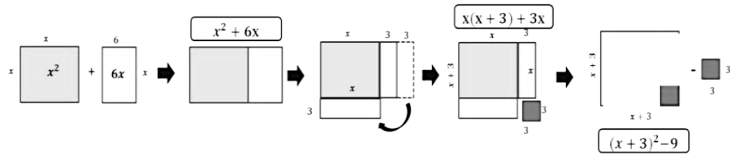Example: Find the roots of the following quadratic equations by the method of completing the square:

i) 2x2 − 7x + 3 = 0

The given quadratic equation is 2x2 − 7x + 3 = 0

The coefficient of x2 is not 1, so we divide the whole equation by 2.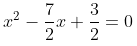Now move 3/2 to RHS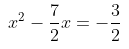Adding (7/4)to both sides we get,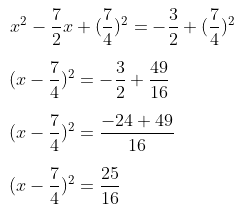Taking square root of both sides we get,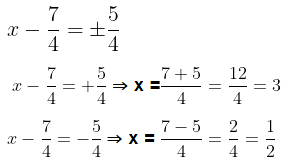The roots of the equation are 3 and 1/2

ii) 4x+ 4√3x + 3 = 0

Dividing the whole equation by 4, so that the coefficient of x2 is 1.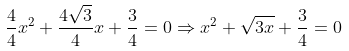Shifting 3/4 to RHS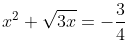Adding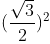to both sides we get,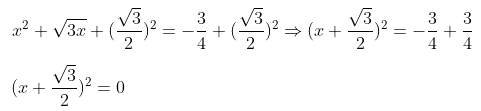Taking the square root of both sides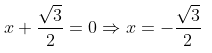The roots of the given equation are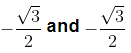x2 − (√5 + 1)x + √5 = 0 by completing the square method.
The given quadratic equation is, x2 − (√5 + 1)x = −√5 = 0

Shifting √5 to RHS we get,

x2 − (√5 + 1)x = −√5
Adding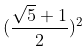to both sides we get,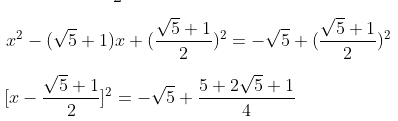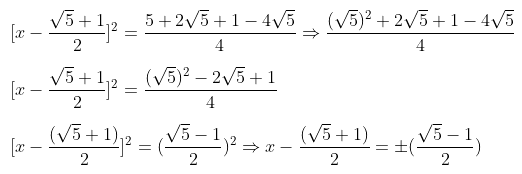Taking +ve sign first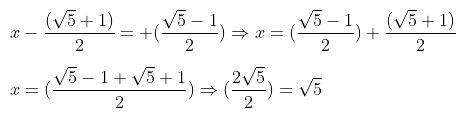Taking –ve sign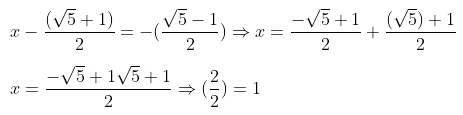The roots of the given equation are √5 and 1

Nature of Roots

The roots of the quadratic equation ax2 + bx + c = 0 are given by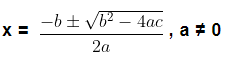Where D = √b2 − 4ac is called the discriminant.

This formula is known as the Quadratic Formula.

The nature of the roots depends upon the value of Discriminant, D.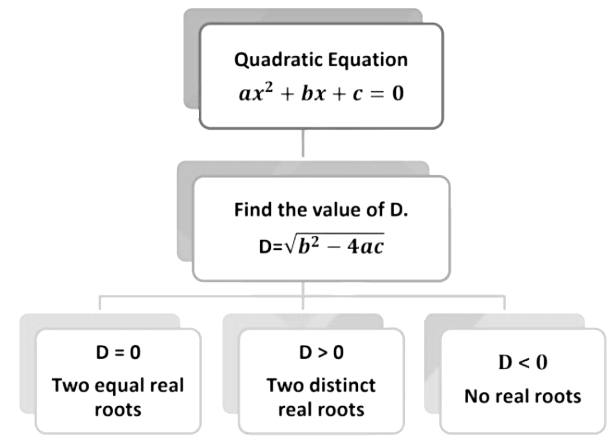Example: Find the roots of the equation,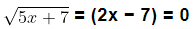The given equation is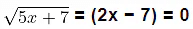Squaring both sides of the equation we get,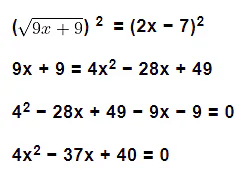Here, a = 4, b = −37 and c = 40

Substituting the value of a, b and c in the quadratic formula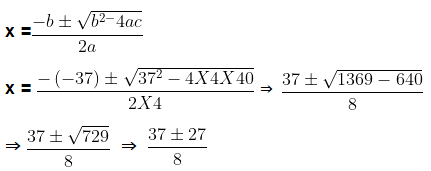Taking +ve sign first,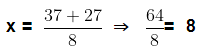Taking -ve we get,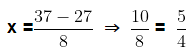The roots of the given equation are 8 and 5/4.

Example: Find the numerical difference of the roots of the equation x2 − 7x − 30 = 0

The given quadratic equation is x− 7x − 30 = 0

Here a = 1, b = −7 and c = −30

Substituting the value of a, b and c in the quadratic formula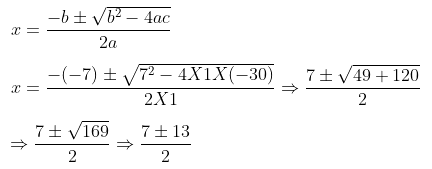Taking +ve sign first,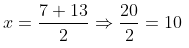Taking -ve we get,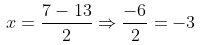The two roots are 10 and -3

The difference of the roots= 10 − (−3) = 10 + 3 = 13

Example: Find the discriminant of the quadratic equation x2 −4x − 5 = 0

The given quadratic equation is x2 − 4x − 5 = 0.

On comparing with ax2 + bx + c = 0 we get,

a = 1,b = −4, and c = −5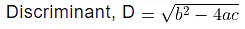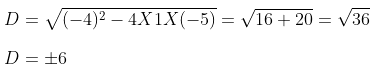Example: Find the value of p, so that the quadratic equation px(x − 2) + 9 = 0 has equal roots.

The given quadratic equation is px(x − 2) + 9 = 0
px2 − 2px + 9 = 0

Now comparing with ax2 + bx + c = 0 we get,

a = p, b = −2p and c = 9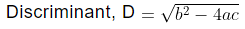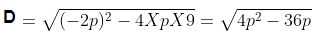The given quadratic equation will have equal roots if D = 0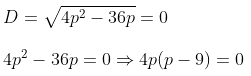p = 0 and p − 9 = 0 ⇒ p = 9
p = 0 and p = 9
The value of p cannot be zero as the coefficient of x, (−2p) will become zero.
Therefore, we take the value of p = 9.

Example: If x = −1 is a root of the quadratic equations 2x2 +px + 5 = 0 and the quadratic equation p(x2 + x) + k = 0 has equal roots, then find the value of k.

The given quadratic equation is 2x2 + px + 5 = 0. If x = −1 is the root of the equation then,
2(−1)2 + p(−1) + 5 = 0
2 − p + 5 = 0 ⇒ −p = −7
p = 7
Putting the value of p in the equation p(x2 + x) + k = 0,
7(x2 + x) + k = 0 ⇒ 7x2 + 7x + k = 0
Now comparing with ax2 + bx + c = 0 we get,
a = 7, b = 7 and c = k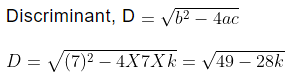The given quadratic equation will have equal roots if D = 0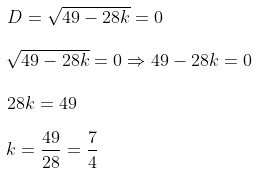Therefore, the value of k is 7/4.

The document Chapter Notes: Quadratic Equations - Notes | Study Mathematics (Maths) Class 10 - Class 10 is a part of the Class 10 Course Mathematics (Maths) Class 10.
All you need of Class 10 at this link: Class 10

## Mathematics (Maths) Class 10

53 videos|403 docs|138 tests
 Use Code STAYHOME200 and get INR 200 additional OFF

## Mathematics (Maths) Class 10

53 videos|403 docs|138 tests

Track your progress, build streaks, highlight & save important lessons and more!

,

,

,

,

,

,

,

,

,

,

,

,

,

,

,

,

,

,

,

,

,

;